# Stress, Satisfaction and Self-Evaluation

This short blog is about exploring the relationships between stress, satisfaction and self-evaluation. For an assignment of the course Introduction to Psychology, I had to gather 20 responses to answer some questions. Due to the huge amount of responses, I thought it could be nice to share the results to thank all of you for your participation.

## Background and hypotheses

The survey, as part of the exercise, was a given fact without any background theory about stress, satisfaction and self-evaluation was supplied. Although we don’t know the background of the survey, we know from prior research about the negative effect of stress on satisfaction (Kaya, Tansey, Melekoglu, & Cakiroglu, 2015).  Besides, we invent some other hypotheses:

• Hypothesis 1: Stress has a negative relation with satisfaction and self-evaluation
• Hypothesis 2: Satisfaction and self-evaluation have a positive relationship
• Hypothesis 3: Age will have a positive relation with satisfaction and self-evaluation
• Hypothesis 4: Gender will moderate the effect of stress on satisfaction and self-evaluation, such that the effect of stress on satisfaction and self-evaluation will higher for women in comparison to men.
• Hypothesis 5: The effect of stress on self-evaluation and satisfaction will decrease over the years.

## METHOD

### Data, Sample, and Procedure

To test our hypotheses, we conducted an online survey. This survey was posted on various social media platforms, and was distributed via email. After a week, reminder emails and posts were created.  We had gender and age as demographic information. We obtained 121 respondents. The mean age of participants was 39.93 years, SD = 14.50, with a range from 18 to 81, of which 53.7% were male.

### Measures

Stress. We used a single-item scale to measure stress and asked participants to rate the item on a 1 (very little stress) to 10 (a lot of stress) Likert scale (M = 5.95, SD = 2.07, min = 1, max = 10). The item was “How much stress do you currently experience in your life?”.

Satisfaction. We used a single-item scale to measure satisfaction and asked participants to rate the item on a 1 (extremely dissatisfied) to 10 (extremely satisfied) Likert scale (M = 7.50, SD = 1.16, min = 3, max = 10). The item was “How satisfied are you currently with your life?”.

Self-Evaluation. We used a single-item scale to measure self-evaluation and asked participants to rate the item on a 1 to 10 (1=low, 10=high) Likert scale (M = 7.55, SD = 1.15, min = 3, max = 10). The item was “If you would have to evaluate yourself, what grade would you give yourself?”.

## RESULTS

Table 1 includes the means, standard deviations, and correlations among the variables in our study. We found a significant negative relationship between stress and satisfaction, as well between stress and self-evaluation, supporting hypothesis 1. Results reveal a significant positive relationship between satisfaction and self-evaluation, supporting hypothesis 2. However, we did not find support for hypothesis 3. Furthermore, we found negative, significant relationships between gender and satisfaction, as well as between gender and self-evaluation, but we don’t know the details of these relationships yet.

To further investigate these differences, we compared both groups. Table 2 shows us the differences between men and women. We see that men on average are nearly 0.5 points more satisfied with their lives, and evaluate themselves also nearly 0.5 points higher. They both experience likewise stress levels. Table 3 explains whether these differences are statistically significant or not. First we observe that the Levene’s test results for both satisfaction as age are significant. That means that the variability in the two groups is not the same for these two variables. So, the scores in one condition vary much more than the scores in your second condition. We could already expect that by looking at the standard deviation for these two variables, where we can see that i.e. the standard deviation of women regarding satisfaction, is higher than the standard deviation of the men’s score. Therefore, we should take the second line of the results to see whether for these variables the groups are significantly different. For the stress and self-evaluation level, we could use the first row of the results. The results show us that there is a significant difference between men (M=7.72, SD=.98) and women (M=7.23, SD=1.31) regarding satisfaction; t(100.61), p= .02. We also found that men (M=7.78, SD=1.01) and women (M=7.29, SD=1.26) differ significantly on their self-evaluation scores; t(119), p=.02, but not regarding stress and age.

Finally, we used the PROCESS macro from Hayes (2012) to analyze the moderating effect of gender on the effect of stress on satisfaction and self-evaluation. As table 4 reveals, we found that stress has a negative significant effect (B = -.12, p = .01) on satisfaction. We also found that gender moderated this effect negatively, but this moderating effect was only significant for women as can be seen in figure 1 and table 5. Please note that for clarification this graph starts as value 5 for satisfaction. These results partly supported hypothesis 4.

When analyzing the moderation of the effect of stress on self-evaluation, we found that stress has a significant negative effect, but the moderating effect of gender was not significant. However, when looking at the details, we found that for men, the effect of stress on self-evaluation was moderated, as can be seen in table 6 and figure 2. These results partly reject hypothesis 4.

Finally, we tested whether the effect of stress on self-evaluation and satisfaction was moderated by age. We controlled for gender as we already found differences in the effects, based on gender. Table 7 reveals the results. We didn’t find support for the moderation of the effect of stress on satisfaction. However, we found that the significance of the negative effect of stress on self-evaluation depended on age. Between age 21 and 46, stress has a significant negative effect on self-evaluation. This effect decreases with age, as can be seen in figure 3. This confirms part of our fifth hypothesis.

## CONCLUSION

Although we missed all basic theoretic knowledge, this study was a nice exercise and revealed some insights, at least for this cross-sectional, convenience sample. The logic relationship between satisfaction and self-evaluation has been confirmed, as well as the idea that men rate themselves higher on both satisfaction and self-evaluation scales. However, the relationship between stress and satisfaction, and stress and self-evaluation highlighted some surprising findings. Stress had a negative, comparable, effect for both men and women regarding self-evaluation, but this effect was only significant for men. On the other hand, we found the opposite for the effect of stress on satisfaction, where the, also stronger, negative effect was only significant for women. Another insight was that age moderated the negative effect of stress on self-evaluation. The effect of stress on self-evaluation stayed negative over the ages, but decreased. Thus, when we are young, or get older, there is still a negative, but decreasing, effect of stress, nonetheless this effect isn’t significant anymore. A possible reason is that this study lacks confounding variables related to the work-life-environment, as the age range where the effect is significant, overlaps with the age range that most people have a job and other duties.

If you want to know more the applied analyses, please feel free to contact me, and if your questions are specifically about graphing the modering effects, you can find more information on posts like graphing conditional indirect effects and graphing moderation.

## References

Kaya, C., Tansey, T., Melekoglu, M., & Cakiroglu, O. (2015). Stress and life satisfaction of Turkish college students. College Student Journal, 257-261.

## Figures and Tables

The images are very small, but clickable to see the details.Figure 1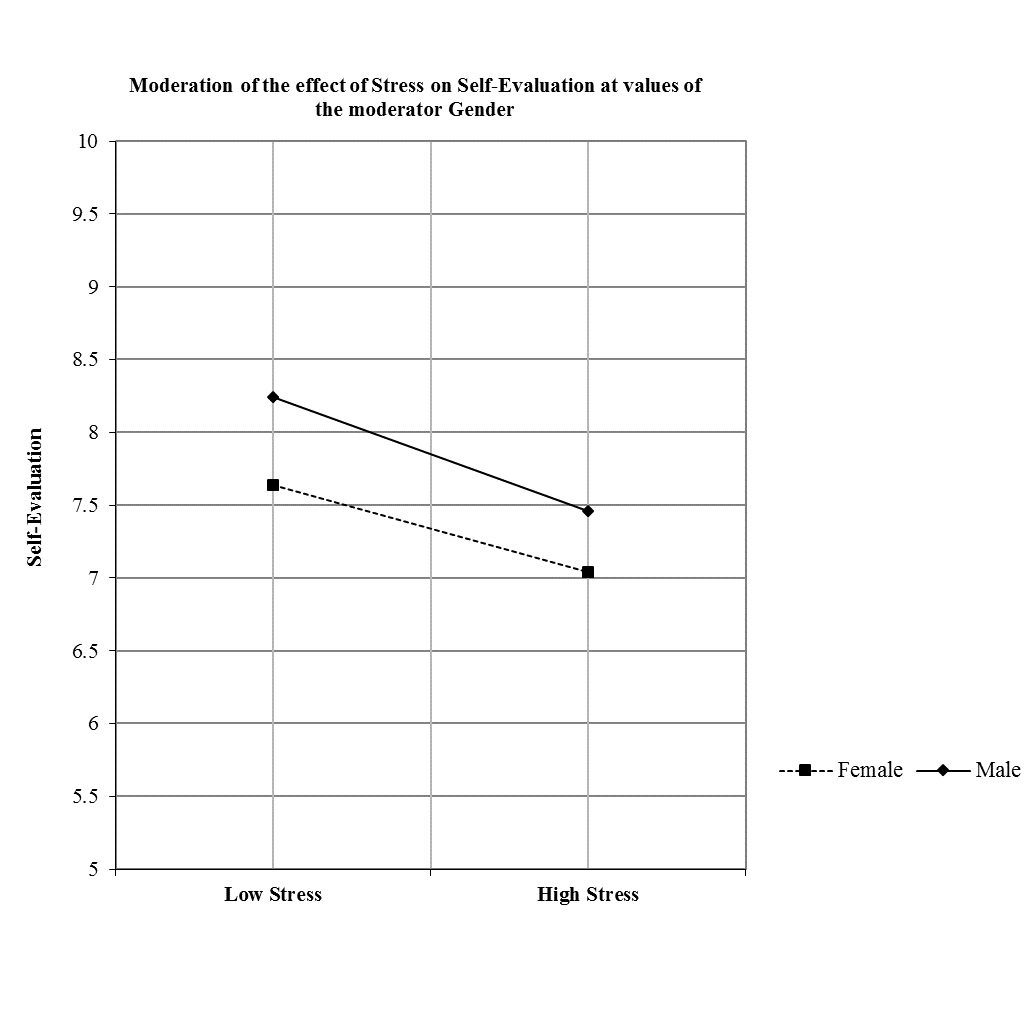Figure 2Figure 3Table 1Table 2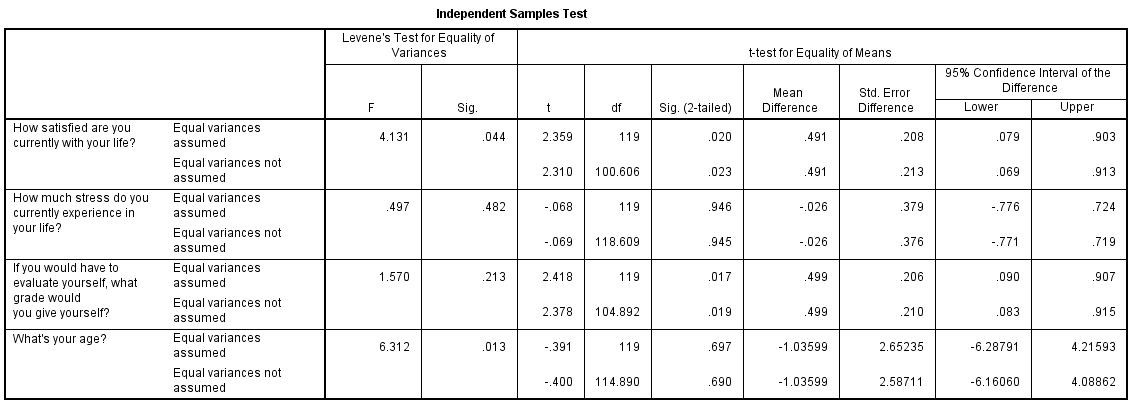Table 3Table 4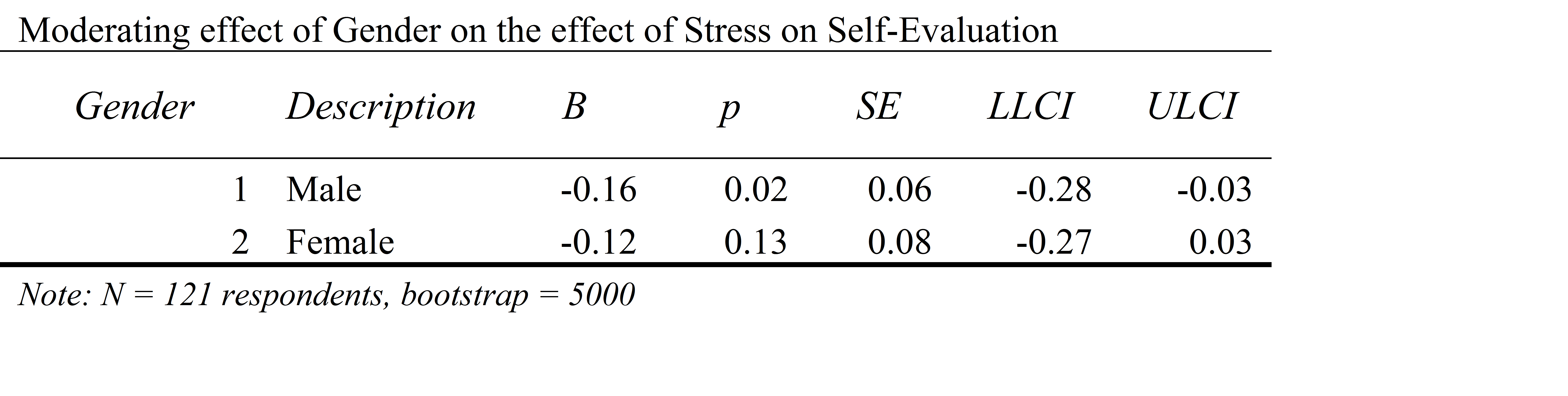Table 5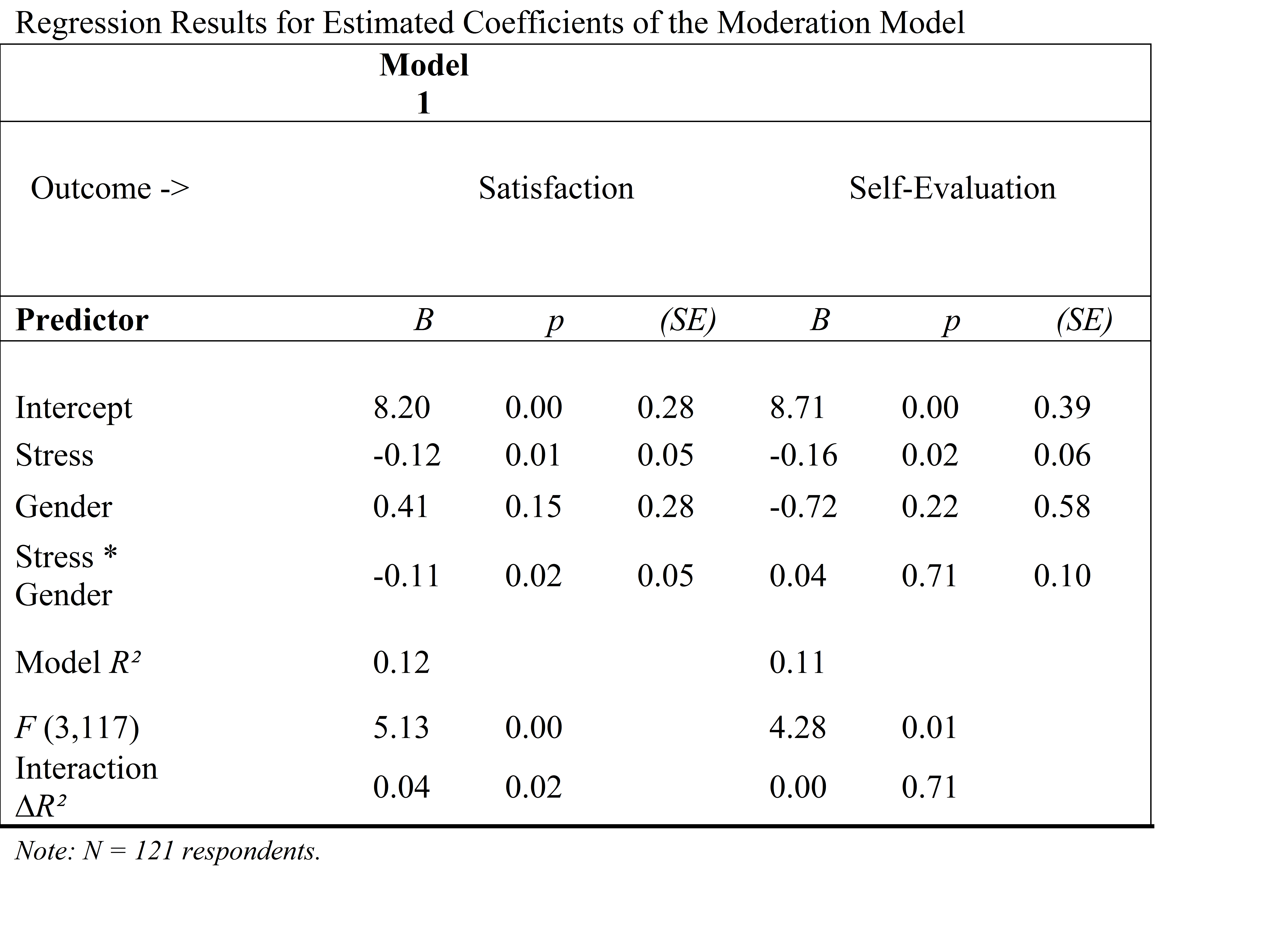Table 6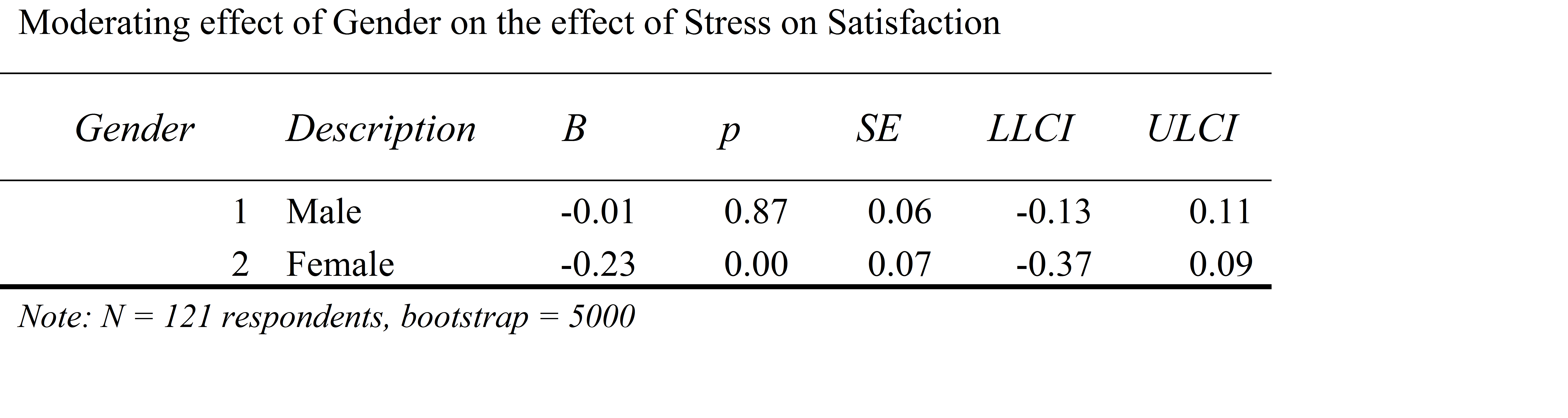Table 7

This site uses Akismet to reduce spam. Learn how your comment data is processed.